# R Vectors

In this tutorial you will learn about the R Vectors and its application with practical example.

## R Vectors

Vector is one of the basic data structure in R. Vector is basically a collection of data elements of the same basic type i.e. logical, integer, double, character, complex or raw. The elements in a vector are termed as components.

## Creating a Vector in R

Vectors are commonly created using the c() function, it is the easiest way to create vectors in R. While, creating vector we must pass elements of the same type, but, if the elements are of different type then elements are converted to the same data type from lower data type to higher data types from logical to integer to double to character.

Example:-

Output:-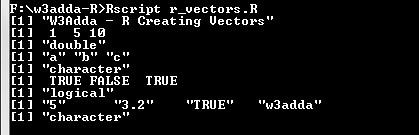Vectors of consecutive or sequential numeric values can simply be generated using colon (:) operator as following –

Syntax:-

start:- It is the start/first element value.
end:- It is the end/last element value.

Example:-

Output:-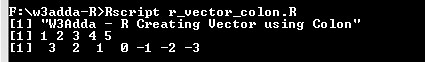## Vector using seq() function

The seq() function enable us to create vectors with sequential values at specified step size.

Syntax:-

Above is the general syntax of seq() function, here

stepSize:- It is step or interval size between vector elements.
startValue:- It is the start value.
endValue:- It is the end value.

Example:-

Output:-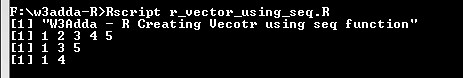## Accessing Vector Elements

Vector elements can be accessed by passing index value(s) in brackets [ ]. An index value can be logical, integer or character.

integer Index:-

An integer index can be used to denote the element position. An integer index value start with 1.

logical Index:-

TRUE, FALSE or 0 and 1 can be used as logical index corresponding to the vector element position, vector with TRUE index position is returned.

character Index:-

Character index vector can be used with named vectors.

Example:-

Output:-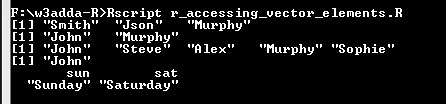## Modifying Vector Elements

Vector element value can be modified by assigning it new value as following –

Example:-

Output:-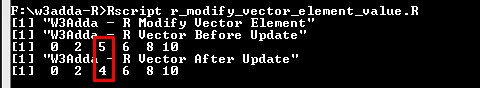## Deleting Vector

Vector can be deleted by simply assigning it NULL value as following –

Example:-

Output:-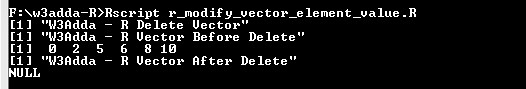In this tutorial we have learn about the R Vectors and its application with practical example. I hope you will like this tutorial.